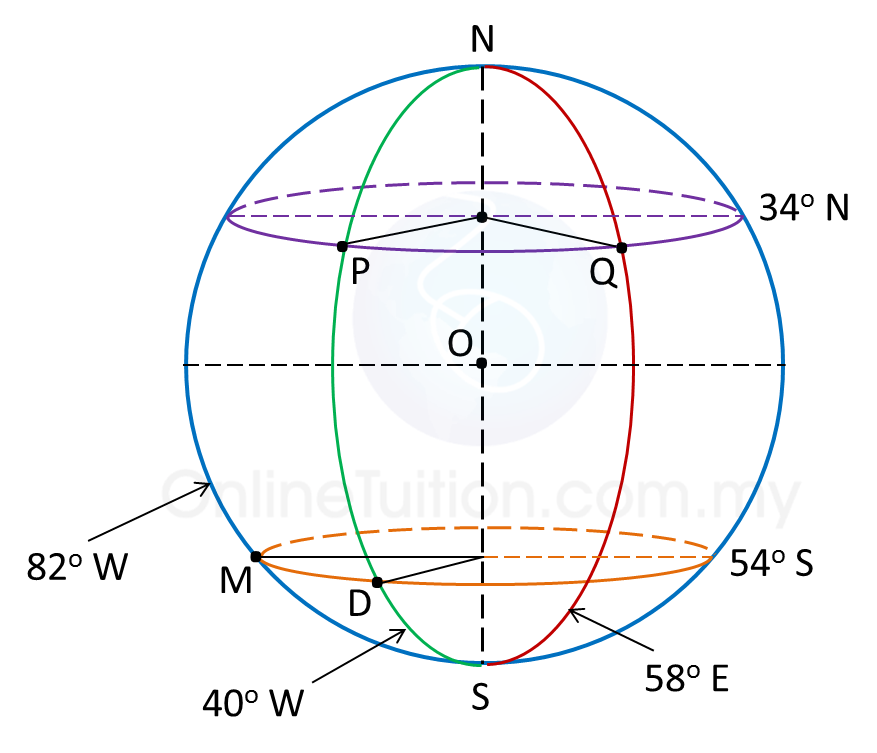# 9.4.2 Distance between Two Points along a Parallel of Latitude

9.4.2 Distance between Two Points along a Parallel of Latitude

1. To find the distance along a parallel of latitude:Distance between points P and Q   = ( different in longitude in minutes ) × cos latitude   = ( θ × 60 × cos xo ) nautical miles

Example:In the diagram above, calculate
(a) The distance of PQ, measured along the parallel of latitude 34o N,
(b) The distance of DM, measured along the parallel of latitude 54o S.

Solution:
(a)
Difference in longitude between P and Q
= 40o + 58o = 98o
Hence, the distance of PQ, measured along the parallel of latitude 34o N
= 98 × 60 × cos 34o← [θ × 60 × cos (latitude)]
= 4874.74 n.m.

(b)
Difference in longitude between D and M
= 82o – 40o = 42o
Hence, the distance of DM, measured along the parallel of latitude 54o S
= 42 × 60 × cos 54o← [θ × 60 × cos (latitude)]
= 1481.22 n.m.